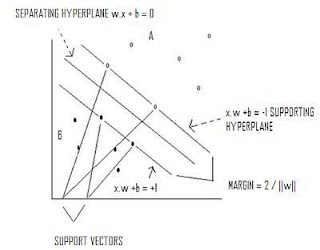## Sunday, September 19, 2010

### Support Vector Machines

Separates classes utilizing supporting hyperplanes and a separating hyperplane that maximizes the margin between classes.Max 2/||w|| s.t. y(x*w+b)-1 >=0

If data is not linearly separable, a kernel function can be used to map data into a space where it is linearly separable.

Input Space →Kernel Function →Feature Space →Input Space.

Quadratic programming provides a decision function:

D(x) = ∑ ∝ k(xi,xj) + b such that if D(x) >0 then x ∊ A

Kernel Functions

Mapping function x→φ(x)

linear kernel

k(xi,xj)=xi'xj

k(xi,xj) = exp[-(||xi –xj||2 / 2 σ2 )]

polynomial kernel

k(xi,xj) = (xixj + a)b

sigmoidal kernel

k(xi ,xj) = tanh(xixj - b)